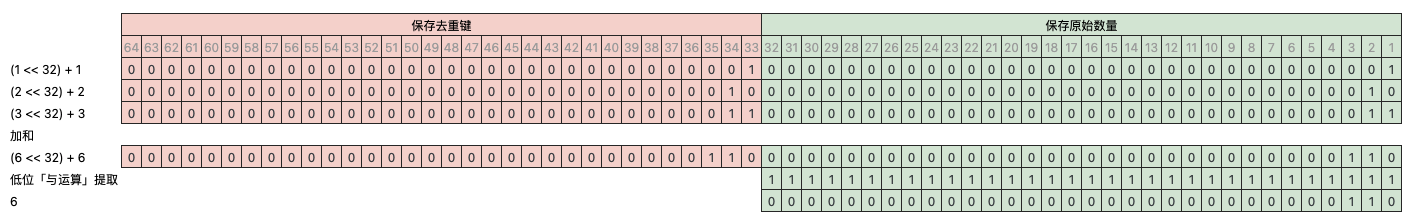# 使用 ClickHouse 做去重加和## 如何做去重加和

• 键鼠套装组合**，组合了一个键盘两个鼠标，商品 ID 为 123，键盘鼠标分别对应库存系统里的两个 SKU JP1 和 SB1
• 键盘 JP1 也单独**，商品 ID 为 456，
• 同样鼠标 SB1 也单独**，商品 ID 为 789

• 订单 o1 买了 1 个 123 键鼠套装
• 订单 o2 买了 2 个 456 键盘
• 订单 o3 买了 1 个 789 鼠标

``````CREATE TABLE f_order
(
`orderID` String,
`productID` UInt32,
`sku` String,
`orgQuantity` UInt16,
`realQuantity` UInt16
)
ENGINE = MergeTree
ORDER BY orderID

INSERT INTO f_order VALUES ('o1', 123, 'JP1', 1, 1), ('o1', 123, 'SB1', 1, 2), ('o2', 456, 'JP1', 2, 2), ('o3', 789, 'SB1', 1, 1)

SELECT *
FROM f_order

*─orderID─*─productID─*─sku─*─orgQuantity─*─realQuantity─*
* o1      *       123 * JP1 *           1 *            1 *
* o1      *       123 * SB1 *           1 *            2 *
* o2      *       456 * JP1 *           2 *            2 *
* o3      *       789 * SB1 *           1 *            1 *
*─────────*───────────*─────*─────────────*──────────────*
``````

orgQurantity 表示原始**数量 realQuantity 表示库存实际扣减的数量

• 统计原始销量汇总为 4（123：1，456：2，789：1）
• 统计商品（productID）维度的原始销量为 123：1，456：2，789：1
• 统计 sku 维度的实际销量为 JP1：3，SB1：3
• 统计 sku 维度的原始销量为 JP1：3，SB1：2

### 常规做法

``````SELECT sum(orgQuantity) AS totalOrgQuantity
FROM
(
SELECT any(orgQuantity) AS orgQuantity
FROM f_order
GROUP BY orderID, productID
)

*─totalOrgQuantity─*
*                4 *
*──────────────────*
``````

### ClickHouse 做法

#### 做法一

``````SELECT arraySum(x -> (x.3), groupUniqArray((orderID, productID, orgQuantity))) AS totalOrgQuantity
FROM f_order

*─totalOrgQuantity─*
*                4 *
*──────────────────*
``````

``````SELECT sum(number) AS x
FROM
(
SELECT number
FROM numbers(10000000)
)

*──────────────x─*
* 49999995000000 *
*────────────────*

1 rows in set. Elapsed: 0.012 sec. Processed 10.02 million rows, 80.18 MB (814.14 million rows/s., 6.51 GB/s.)

SELECT arraySum(x -> x, groupUniqArray(number)) AS x
FROM numbers(10000000)

*──────────────x─*
* 49999995000000 *
*────────────────*

1 rows in set. Elapsed: 1.264 sec. Processed 10.02 million rows, 80.18 MB (7.93 million rows/s., 63.45 MB/s.)
``````

#### 做法二

``````-- 将 orgQuantity 修改为 Tuple(k Nullable(UInt32), q UInt16) 类型
-- 第一位 k 作去重键，取值 NULL 表示非组合商品，有值时为组合商品当前订单的订单号和商品 ID 的 crc32 值
CREATE TABLE f_order_2
(
`orderID` String,
`productID` UInt32,
`sku` String,
`orgQuantity` Tuple(k Nullable(UInt32), q UInt16),
`realQuantity` UInt16
)
ENGINE = MergeTree
ORDER BY orderID
SETTINGS index_granularity = 8192

-- 从 f_order 中导入数据，仅 productID 为 123 组合商品时才赋值给 orgQuantity.k
INSERT INTO f_order_2 SELECT
orderID,
productID,
sku,
(if(productID = 123, crc32(concat(orderID, toString(productID))), NULL), orgQuantity),
realQuantity
FROM f_order

SELECT *
FROM f_order_2

*─orderID─*─productID─*─sku─*─orgQuantity────*─realQuantity─*
* o1      *       123 * JP1 * (3806212741,1) *            1 *
* o1      *       123 * SB1 * (3806212741,1) *            2 *
* o2      *       456 * JP1 * (NULL,2)       *            2 *
* o3      *       789 * SB1 * (NULL,1)       *            1 *
*─────────*───────────*─────*────────────────*──────────────*
``````

``````SELECT arraySum(x -> (x.2), groupUniqArrayIf(orgQuantity, isNotNull(orgQuantity.k))) + sumIf(orgQuantity.q, isNull(orgQuantity.k)) AS totalOrgQuantity
FROM f_order_3

*─totalOrgQuantity─*
*                4 *
*──────────────────*
``````

#### 做法三``````-- 将 orgQuantity 修改为 UInt64 类型
-- 高位 33 ~ 64 用来保存「去重键」, 为 0 表示非组合商品，有值时为组合商品当前订单的订单号和商品 ID 的 crc32 值
CREATE TABLE f_order_3
(
`orderID` String,
`productID` UInt32,
`sku` String,
`orgQuantity` UInt64,
`realQuantity` UInt16
)
ENGINE = MergeTree
ORDER BY orderID
SETTINGS index_granularity = 8192

-- 从 f_order 中导入数据，仅 productID 为 123 组合商品时才赋值给 orgQuantity.k
INSERT INTO f_order_3 SELECT
orderID,
productID,
sku,
bitShiftLeft(toUInt64(if(productID = 123, crc32(concat(orderID, toString(productID))), 0)), 32) + orgQuantity,
realQuantity
FROM f_order

SELECT *
FROM f_order_3

*─orderID─*─productID─*─sku─*──────────orgQuantity─*─realQuantity─*
* o1      *       123 * JP1 * 16347559244213518337 *            1 *
* o1      *       123 * SB1 * 16347559244213518337 *            2 *
* o2      *       456 * JP1 *                    2 *            2 *
* o3      *       789 * SB1 *                    1 *            1 *
*─────────*───────────*─────*──────────────────────*──────────────*
``````

``````-- 统计原始销量汇总为 4（123：1，456：2，789：1）
SELECT bitAnd(sumDistinctIf(orgQuantity, orgQuantity >= 4294967296), 4294967295) + sumIf(orgQuantity, orgQuantity < 4294967296) AS totalOrgQuantity
FROM f_order_3

*─totalOrgQuantity─*
*                4 *
*──────────────────*

-- 统计商品（productID）维度的原始销量为 123：1，456：2，789：1
SELECT
productID,
bitAnd(sumDistinctIf(orgQuantity, orgQuantity >= 4294967296), 4294967295) + sumIf(orgQuantity, orgQuantity < 4294967296) AS totalOrgQuantity
FROM f_order_3
GROUP BY productID

*─productID─*─totalOrgQuantity─*
*       456 *                2 *
*       789 *                1 *
*       123 *                1 *
*───────────*──────────────────*

-- 统计 sku 维度的实际销量为 JP1：3，SB1：3
SELECT
sku,
sum(realQuantity) AS totalRealQuantity
FROM f_order_3
GROUP BY sku

*─sku─*─totalRealQuantity─*
* JP1 *                 3 *
* SB1 *                 3 *
*─────*───────────────────*

-- 统计 sku 维度的原始销量为 JP1：3，SB1：2
SELECT
sku,
bitAnd(sumDistinctIf(orgQuantity, orgQuantity >= 4294967296), 4294967295) + sumIf(orgQuantity, orgQuantity < 4294967296) AS totalOrgQuantity
FROM f_order_3
GROUP BY sku

*─sku─*─totalOrgQuantity─*
* JP1 *                3 *
* SB1 *                2 *
*─────*──────────────────*
``````

#### 小结

``````CREATE TABLE x_tuple
(
`x` Tuple(Nullable(UInt32), UInt16)
)
ENGINE = MergeTree
ORDER BY x

-- 模拟 9 / 10 的数据为非组合商品（没有去重键）
INSERT INTO x_tuple SELECT x
FROM
(
SELECT
number % 100000 AS y,
(if(y > 90000, NULL, y), (number % 4) + 1) AS x
FROM numbers(10000000)
)

SELECT arraySum(x -> (x.2), groupUniqArrayIf(x, isNotNull(x.1))) + sumIf(x.2, isNull(x.1)) AS x
FROM x_tuple

*───────x─*
* 2724901 *
*─────────*

1 rows in set. Elapsed: 0.381 sec. Processed 10.00 million rows, 70.00 MB (26.26 million rows/s., 183.81 MB/s.)
``````

``````CREATE TABLE x_distinct
(
`x` UInt64
)
ENGINE = MergeTree
ORDER BY x

-- 模拟 9 / 10 的数据为非组合商品（没有去重键）
INSERT INTO x_distinct SELECT x
FROM
(
SELECT
number % 100000 AS y,
(if(y > 90000, 0, bitShiftLeft(toUInt64(y + 1), 32)) + (number % 4)) + 1 AS x
FROM numbers(10000000)
)

SELECT bitAnd(sumDistinctIf(x, x >= 4294967296), 4294967295) + sumIf(x, x < 4294967296) AS x
FROM x_distinct

*───────x─*
* 2724901 *
*─────────*

1 rows in set. Elapsed: 0.170 sec. Processed 10.00 million rows, 80.00 MB (58.71 million rows/s., 469.69 MB/s.)
``````